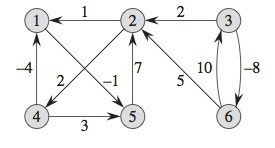# Design and Analysis of Algorithms

July 17, 2019

## Problem of Finding All Pairs of Shortest Path

• Chapter 25 of Introduction to Algorithms (3rd Edition), Thomas H. Cormen, Charles E. Leiserson, Ronald L. Rivest, and Clifford Stein.

• Find the shortest paths between all pairs of vertices in a graph
• The problem to make a distances table between all pairs of cities in a Roads Atlas

## Problem of Finding All Pairs of Shortest Path

• We start with a directed and weighted graph $$G = (V, E)$$ with a weights function $$w: E \rightarrow R$$ that maps edges to weights with real values
• Find for each pair of vertices $$u$$, $$v \in V$$
• A shortest path (with the lowest weight) from $$u$$ to $$v$$
• The weight of the path is the sum of the weights of its edges
• Output in tabular form
• Entry in row $$u$$ and column $$v$$ is the weight of the shortest path between $$u$$ and $$v$$

## Modeling the Problem

• Weighted and directed graph, $$G = (V, E)$$
• Weights: $$W = (w_{ij})$$

$$\delta w_{i,j} = \begin{cases} 0 &\mbox{if } i = j\\ w_{i,j} &\mbox {if } i \not= j, (i,j) \in E\\ \infty &\mbox{if } i \not= j, (i,j) \notin E\\ \end{cases}$$

• Negative weight edges are allowed
• The input graph does not contain negative weight cycles

## Modeling the Problem

• Tabular output of all pairs of shortest paths
• $$n$$ x $$n$$ matrix, $$D = (d_{i,j})$$
• $$d_{i,j}$$ contains the weight of the shortest path from vertex $$i$$ to $$j$$
• $$\delta(i, j)$$ denotes the weight of the shortest path from vertex $$i$$ to $$j$$
• At termination time of the algorithm $$d_{i,j} = \delta(i, j)$$
• We also compute a predecessors matrix
• $$\Pi = (\pi_{i,j})$$, with value NIL if $$i = j$$ or if there is not a path from $$i$$ to $$j$$ and in other case $$\pi_{i,j}$$ is a predecessor of $$j$$ for some path, shorter from $$i$$

## Modeling the Problem

• Predecessor Subgraph
• The subgraph induced by the $$i^{th}$$ row of matrix $$\Pi$$ must be a tree of shortest paths with root in $$i$$
• The predecessor subgraph of $$G$$ for $$i$$ is defined as $$G_{\pi,i} = (V_{\pi,i}, E_{\pi,i})$$, where
• $$V_{\pi,i} = \{j \in V: \pi_{i,j} \neq NIL\} \cup \{i\}$$, and
• $$E_{\pi,i} = \{(\pi_{i,j}, j) : j \in V_{\pi,i} - \{i\}\}$$.

## Shortest Paths and Matrix Multiplication

• Dynamic programming solution for the problem of all pairs of shortest paths with a directed graph $$G = (V, E)$$
• Calls function similar to matrix multiplication
• First algorithm, $$\Theta(V^4)$$
• Enhancement in $$\Theta(V^3lgV)$$

## Dynamic Programming Reminder

• 4 steps
• Characterize the structure of an optimal solution
• Recursively define the value of an optimal solution
• Compute the value of the optimal solution in an bottom-up way
• Build the optimal solution with the computed information

## Optimum Substructure of a Shortest Path - Proof to lemma 24.1

• Decompose path $$p$$ in $$v_1 \overset{p_{1i}}{\longrightarrow} v_i \overset{p_{ij}}{\longrightarrow} v_j \overset{p_{jk}}{\longrightarrow} v_k$$
• We then have that $$w(p) = w(p_{1i}) + w(p_{ij}) + w(p_{jk})$$
• We assume that there is a path $$p_{ij}\prime$$ from $$v_i$$ to $$v_j$$ with weight $$w(p_{ij}^\prime) < w(p_{ij})$$
• Then the path from $$v_1$$ to $$v_k$$ that passes through $$p_{ij}^\prime$$ $$v_1 \overset{p_{1i}}{\longrightarrow} v_i \overset{p_{ij}^\prime}{\longrightarrow} v_j \overset{p_{jk}}{\longrightarrow} v_k$$ with weight $$w(p_{1i}) + w(p_{ij}^\prime) + w(p_{jk})$$ has a weight lower than $$w(p)$$
• This is a contradiction to our assumption, that $$p$$ is a shortest path from $$v_1$$ to $$v_k$$

## Recursive Solution for All Pairs of Shortest Paths

• Let $$l_{ij}^{(m)}$$ be the minimum weight of a path from vertex $$i$$ to $$j$$ that has at least $$m$$ edges
• When $$m = 0$$, there is a shortest path from $$i$$ to $$j$$ with no edges iff $$i = j$$, then

$$l_{ij}^{(0)} = \begin{cases} 0 &\mbox{if } i = j,\\ \infty &\mbox{if } i \not= j.\\ \end{cases}$$

## Recursive Solution for All Pairs of Shortest Paths

• For $$m \ge 1$$, we compute $$l_{ij}$$ as the minimum of $$l_{ij}^{(m-1)}$$ (path from $$i$$ to $$j$$ with at most $$m-1$$ edges) and the minimum weight of the path from $$i$$ to $$j$$ with at most $$m$$ edges
• With all possibles predecessors $$k$$ of $$j$$

$$l_{ij}^{(m)} = \min(l_{ij}^{(m-1)}, \min_{1 \leq k \leq n}\{l_{ik}^{(m-1)} + w_{kj}\})$$ $$= \min_{1 \leq k \leq n}(l_{ik}^{(m-1)} + w_{kj})$$

• The weights of the shortest path are given by
• $$\delta(i, j) = l_{ij}^{(n-1)} = l_{ij}^{(n)} = l_{ij}^{(n+1)} = \dots$$
• There are at most $$n - 1$$ edges in the shortest path from $$i$$ to $$j$$ assuming that there are no cycles with negative weight

## Computing All Weights of the Shortest Path - Bottom-UP

• We take as input matrix $$W = (w_{ij})$$
• Compute matrices
• $$L^{(1)}$$, $$L^{(2)}$$, $$\dots$$, $$L^{(n-1)}$$, where for $$m = 1, 2, ..., n - 1$$ we have that $$L^{(m)} = (l_{ij}^{(m)})$$
• The final matrix has the weights of the shortest paths
• $$l_{ij}^{(1)} = w_{ij}$$ for all vertices $$i$$, $$j \in V$$, then $$L^{(1)} = W$$

## Algorithm

• Given matrices $$L^{(m-1)}$$ and $$W$$, it returns $$L^{(m)}$$
• It extends the shortest paths obtained up to now by adding an edge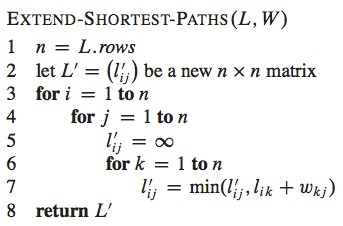## Algorithm

• The algorithm is based on the recursive equation
• Execution time of $$\Theta(n^3)$$ for the nested loops
• Similar to matrix multiplication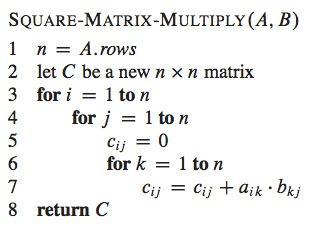## Algorithm

• Algorithm to find all pairs of shortest paths
• Based on Extend-Shortest-Paths
• This algorithm is executed in time $$\Theta(n^4)$$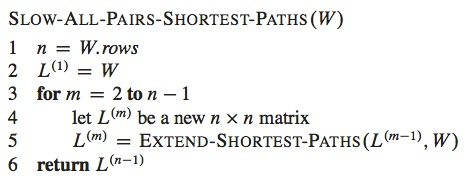## Example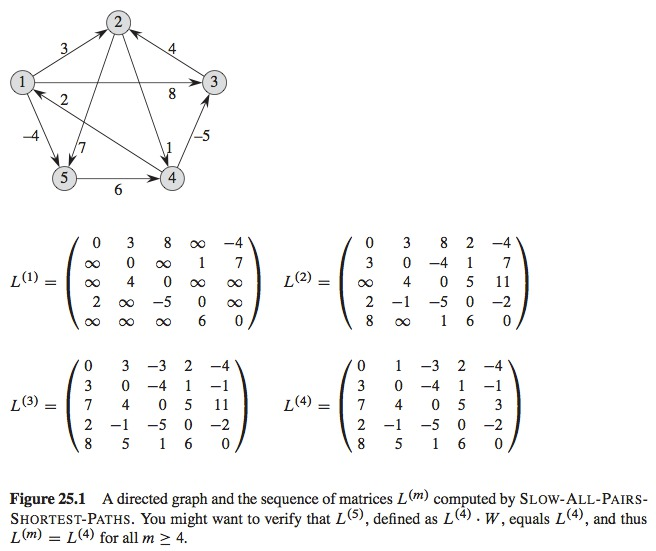## Faster Algorithm

• We don’t want to compute $$L^{(m)}$$ because we have the result from $$L^{(n-1)}$$, assuming that there are no cycles with negative weight, $$L^{(m)} = L^{(n-1)}$$ in $$\lceil(lg(n-1))\rceil$$ with the sequence: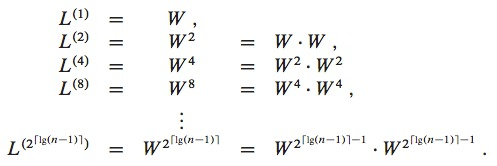• Keep going up to $$L^{(2\lceil lg(n-1 )\rceil)}$$
• This process is known as the repeating squaring technique
• It requires only $$\lceil lg(n-1) \rceil$$ matrices products, calls to EXTEND-SHORTEST-PATHS

## Faster Algorithm

• Execution time of $$\Theta(n^3lgn)$$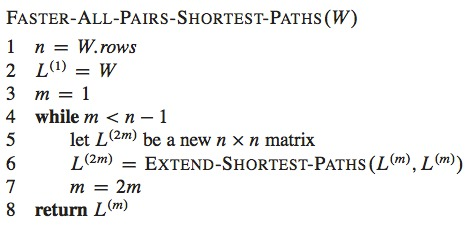## Floyd-Warshall Algorithm

• Uses a different dynamic programming approach
• Execution time of $$\Theta(V^3)$$
• Accepts negative weight edges but not negative weight cycles
• We follow the dynamic programming process to develop the algorithm

## Floyd-Warshall Algorithm

• The structure of a shortest path
• Considers the intermediate vertices of a shortest path
• If $$p = \langle v_1, v_2, \dots, v_l \rangle$$
• $$v_2 \dots v_{l-1}$$ are the intermediate vertices
• The Floyd-Warshall algorithm works by sucessively reducing the number of intermediate vertices that may occur in a shortest path and its subpaths
• Let $$G = (V, E)$$ be the graph with vertices $$V$$ numbered from $$1 \dots n$$
• Consider a subset of vertices $$\{1, 2, \dots k\}$$ for some $$k$$
• Consider all paths from $$i$$ to $$j$$ with intermediate vertices taken from $$\{1, 2, \dots, k\}$$
• Let $$p$$ be the minimum weight of the path from them
• One of two situations may occur:

## Floyd-Warshall Algorithm

1. $$k$$ is not an intermediate vertex of $$p$$
• $$i \overset{p}{\longrightarrow} j$$
2. $$k$$ is an intermediate vertex of $$p$$
• $$i \overset{p_1}{\longrightarrow} k \overset{p_2}{\longrightarrow} j$$
• Contains vertices from $$\{1, 2, \dots, k-1\}$$
• $$p_1$$ is the shortest path from $$1$$ to $$k$$
• $$p_2$$ is the shortest path from $$k$$ to $$j$$
• This is because of lemma 24.1

## Recursive Solution

• Let $$d_{ij}^{(k)}$$ be the weight of the shortest path from $$i$$ to $$j$$ with all intermediate vertices in $$\{1, 2, \dots, k\}$$
• Because for each path, intermediate vertices are in the set $$\{1, 2, \dots, n\}$$, matrix $$D^{(n)} = (d_{ij}^{(n)})$$ will contain the final solution $$\delta(i, j)$$ for each $$i, j \in V$$.
• Recurrence: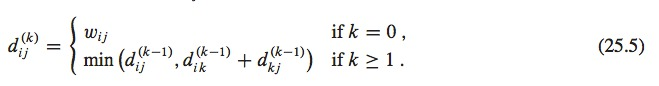## Floyd-Warshall Algorithm

• Computes $$d_{ij}^{(k)}$$ values in growing order of the values of $$k$$
• Input: $$n$$ x $$n$$ matrix $$W$$
• Returns $$D^{(n)}$$ with the weights of the shortest path
• Execution time $$\Theta(n^3)$$, better than the previous ones with $$O(n^3lgn)$$ and $$O(n^4)$$

## Floyd-Warshall Algorithm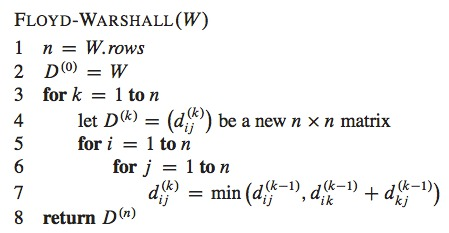## Example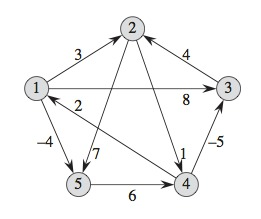## Example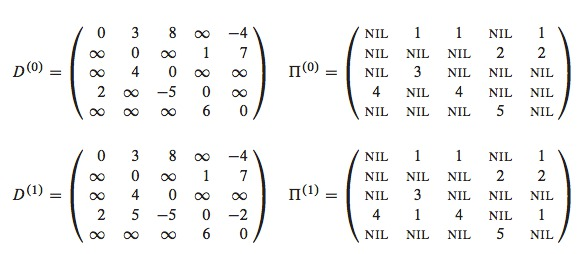## Example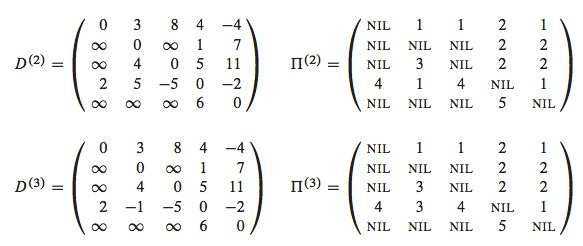## Example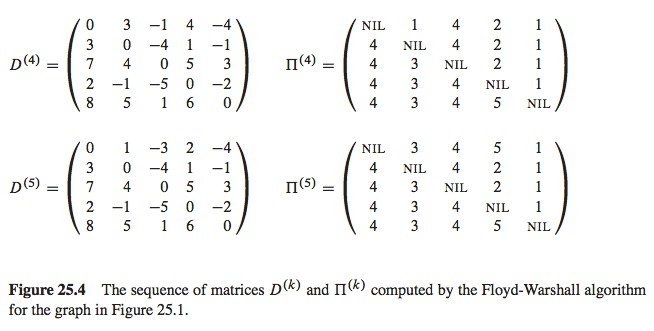## Construction of the Shortest Path

• There are several methods to build the paths with the Floyd-Warshall algorithm
• Construct matrix $$D$$ of weights of shortest paths and then the predecessors matrix $$\Pi$$ from $$D$$
• Construct the predecessors matrix on-line, according to the Floyd-Warshall’s algorithm it builds $$D^{(k)}$$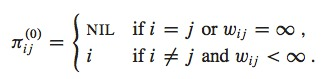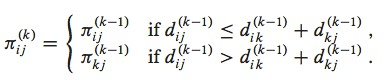## Exercise

• Use the Floyd Warshall algorithm with the following graph